Spectrum of an element

The set of numbers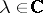for which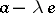is non-invertible (the algebra is assumed to be complex,is a given element of it andis the identity of the algebra). The spectrum is a non-empty compact set (the Gel'fand–Mazur theorem). In the case of a commutative algebra, the spectrum coincides with the set of values on this element of all the characters of the algebra (cf. Character of a-algebra).
This concept can be used as a basis for developing a functional calculus for the elements of a Banach algebra. The natural calculus of polynomials in an elementof a Banach algebrais extended to a continuous homomorphism intofrom the ring of germs of functions holomorphic in a neighbourhood of the spectrum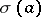. The necessity of considering functions in several variables leads to the concept of the joint spectrum of a system of elements of a Banach algebra. Ifis commutative, then, by definition, the spectrum of a set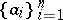of elements inis the collectionof all-tuples of the form, whereis a character of. In general, one defines the left (right) spectrum ofto include those sets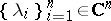for which the system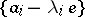is contained in a non-trivial left (respectively, right) ideal of the algebra. The spectrum is then defined as the union of the left and right spectra. For the basic results of multi-parametric spectral theory, and also for other approaches to the concept of the spectrum of a set of elements, see .